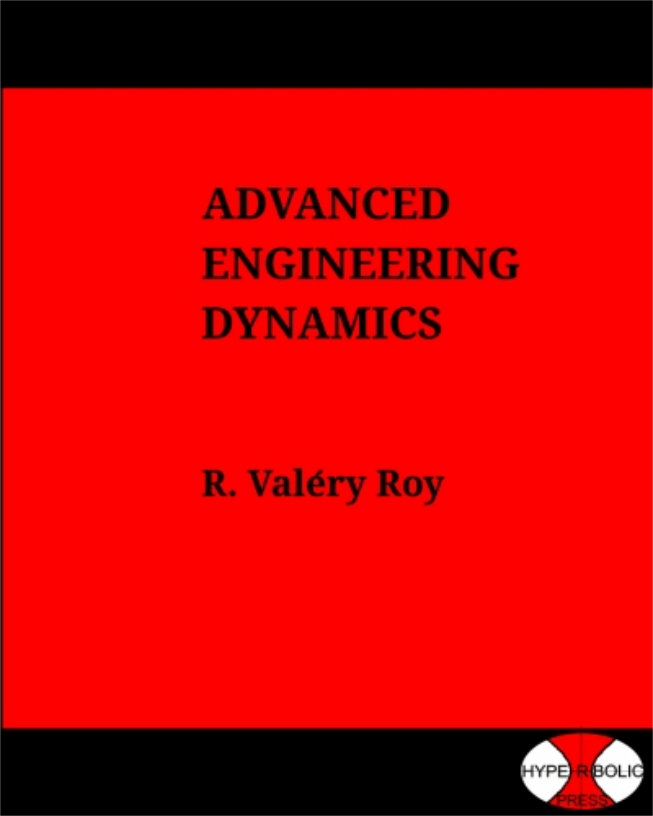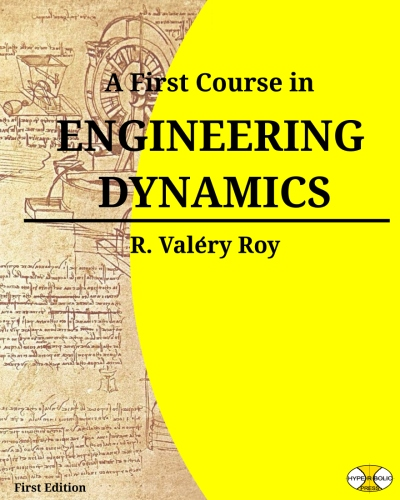# Published Books

## ISBN-13: 978-0990696926 (b & w), AmazonAdvanced Engineering Dynamics was written for graduate students and research scientists in Mechanical Engineering. It covers a wide range of fundamental and advanced topics of engineering dynamics usually not found in a single tome. It is written in a compact, concise and rigorous style. The methods, tools and notations advocated in this book will appear to be novel to most readers. They hinge upon the use of mathematical objects called screws. Screws provide a simple yet powerful formalism which unifies all aspects of rigid body mechanics. Each chapter is illustrated by many examples which are essential to full comprehension of the subject. This book will be useful to a wide range of fields of application, such as robotics, spacecraft mechanics, or biomechanics.

Content: Chapter 1: Position & Displacement. Chapter 2: Particle Kinematics. Chapter 3: Rigid Body Kinematics. Chapter 4: Screw Theory. Chapter 5: Kinematic Screw of a Rigid Body. Chapter 6: Relative Motion Analysis. Chapter 7: Kinematics of Constrained Bodies. Chapter 8: Kinematic Analysis of Mechanisms. Chapter 9: Mass Distribution. Chapter 10: Mechanical Actions. Chapter 11: Newton-Euler Formalism. Chapter 12:Power, Work & Energy. Chapter 13: Lagrange Equations. Chapter 14: Gibbs-Appell & Kane Equations. Chapter 15: Gyroscopic Phenomena. Chapter 16: Non-Newtonian Referentials.

## color: ISBN 0990696987, AmazonA First Course in Engineering Dynamics (2nd Edition) covers basic topics typically taught in a second mechanics course in a two-course sequence in most mechanical engineering curricula. Engineering Dynamics is a scientific branch of classical mechanics with a long and rich history. Despite the fact that its foundations were set long ago, it is a continuously evolving discipline, as it is the driving force behind the research and development of many technological applications.

The book is structured in two parts and six chapters:

Part I: Kinematics, Chapters 1 and 2, Part II: Kinetics, Chapters 3 through 6.

The first two chapters are devoted to Kinematics, the branch of mechanics which deals with the study of motions without consideration of their causes. Kinematics is first applied to particle motion (Chapter 1), then to rigid body motion (Chapter 2), where more realistic engineering models are studied. Part II deals with Kinetics, that is, the branch of mechanics which studies the relationships between motion and the cause of motion. It is comprised of four chapters, each presenting a specific formulation of kinetics laws: Chapter 3, Linear Momentum Formulation, Chapter 4, Angular Momentum Formulation, Chapter 5, Energy Formulation, Chapter 6, Impulse/Momentum Formulation. This structure offers many advantages, in that it allows presentations and proper justification of many fundamental topics usually not found in standard textbooks. The style of this book is concise and mathematical. It provides students with an opportunity to learn how to describe a physical phenomenon in the language of mathematics. With Dynamics, students will learn to develop a systematic modeling method to transform a physical system into a mathematical representation. As Galileo put it, “The book of Nature is written in mathematical language.”